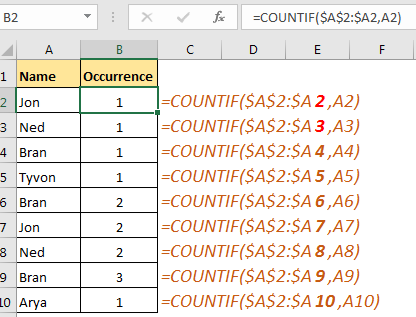# Expanding References in Excel

The ranges that expand when copied in the below cells or to right cells are called expanding references.
Where do we need expanding references? Well, there were many scenarios where I needed them but for this article, I am taking this simple example.

Example 1:

Here, I have a list of names. Now I want to mark the occurrence of each name in the range. I mean, on the first occurrence of Jon, I want to mark 1, on the second occurrence of Jon, I want to mark it as 2 and so on. I want to do this for each name in the range.

To do so, I use a COUNTIF formula:

=COUNTIF(\$A\$2:\$A2,A2)You can see that it numbers each occurrence of each name.

How Does It Work?
If you want your range to expand downward, use this method.
Make the first part of the range Absolute. In the second part, make column absolute and leave only row numbers open (\$A\$2:\$A2).

\$col\$row:\$colrow

This will lock everything in and will allow the row to change relatively when copied in the below cells.

In the above example, we have done this with range \$A\$2:\$A2. The COUNTIF function is counting A2 in this range. Now when you’ll copy this formula in the below cells, it will change to \$A\$2:\$A3, \$A\$2:\$A4 and so on. The COUNTIF function will return count of value in the relative cell, in the expanded list.

Similarly, if you want your range to expand horizontally, leave the column relative and make the row absolute in the second part of the range.

\$col\$row:col\$row

This will allow your range to expand horizontally while copying to the right of the cell.

So yeah guys, this was a quick tutorial about expanding references. Let me know if this was helpful in the comments section below. I have mentioned some related and popular article on Excel 2016. Check'em out.

Related Articles:

Dynamic Worksheet Reference in Excel

What are Relative and Absolute Reference in Excel

Perform Shortcut To Toggle Between Absolute and Relative References in Excel

How to Count Unique Values In Excel

All About Excel Named Ranges in Excel

Popular Articles

50 Excel Shortcut to Increase Your Productivity : Get faster at your task. These 50 shortcuts will make you work even faster on Excel.

How to use the VLOOKUP Function in Excel : This is one of the most used and popular functions of excel that is used to lookup value from different ranges and sheets.

How to use the COUNTIF function in Excel : Count values with conditions using this amazing function. You don't need to filter your data to count specific values. Countif function is essential to prepare your dashboard.

How to use the SUMIF Function in Excel : This is another dashboard essential function. This helps you sum up values on specific conditions.

Terms and Conditions of use

The applications/code on this site are distributed as is and without warranties or liability. In no event shall the owner of the copyrights, or the authors of the applications/code be liable for any loss of profit, any problems or any damage resulting from the use or evaluation of the applications/code.